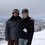# Happy Easter! - 2

I tried to write this problem, and I've realized I've completely gone off the tracks.

If we randomly scramble the letters of HAPPYEASTER, in how many ways can exactly 2 sets of the duplicated letters appear next to each other in the string?

Example of desired outcome:

AAPYHSPEERTNote by Eric Roberts
3 weeks, 5 days ago

This discussion board is a place to discuss our Daily Challenges and the math and science related to those challenges. Explanations are more than just a solution — they should explain the steps and thinking strategies that you used to obtain the solution. Comments should further the discussion of math and science.

When posting on Brilliant:

• Use the emojis to react to an explanation, whether you're congratulating a job well done , or just really confused .
• Ask specific questions about the challenge or the steps in somebody's explanation. Well-posed questions can add a lot to the discussion, but posting "I don't understand!" doesn't help anyone.
• Try to contribute something new to the discussion, whether it is an extension, generalization or other idea related to the challenge.

MarkdownAppears as
*italics* or _italics_ italics
**bold** or __bold__ bold
- bulleted- list
• bulleted
• list
1. numbered2. list
1. numbered
2. list
Note: you must add a full line of space before and after lists for them to show up correctly
paragraph 1paragraph 2

paragraph 1

paragraph 2

[example link](https://brilliant.org)example link
> This is a quote
This is a quote
    # I indented these lines
# 4 spaces, and now they show
# up as a code block.

print "hello world"
# I indented these lines
# 4 spaces, and now they show
# up as a code block.

print "hello world"
MathAppears as
Remember to wrap math in $$ ... $$ or $ ... $ to ensure proper formatting.
2 \times 3 $2 \times 3$
2^{34} $2^{34}$
a_{i-1} $a_{i-1}$
\frac{2}{3} $\frac{2}{3}$
\sqrt{2} $\sqrt{2}$
\sum_{i=1}^3 $\sum_{i=1}^3$
\sin \theta $\sin \theta$
\boxed{123} $\boxed{123}$

Sort by:

Well, here's my brute-force solution. :) Running the following code (which could take around a minute due to the $\dfrac{11!}{2!2!2!} = 4,989,600$ initial permutations) yields a total number of ways of $\boxed{423,360}$ which is $\dfrac{14}{165}$ of the original permutations (for as yet unknown reasons).

  1 2 3 4 5 6 7 8 9 10 11 12 13 14 15 16 17 18 19 20 21 22 23 24 25 26 # we'll need this for later from itertools import permutations as perms # create a list of all the letters in "Happy Easter" letters = [l for l in 'HAPPYEASTER'] # create a list with itertools.permutations (imported as perms) of all possible permutations of the letters # we also convert it to a set to remove duplicate permutations (since AA for instance will be counted twice) letter_perms = set([p for p in perms(letters)]) # start counting permutations which meet our criteria valid_perms = 0 # loop through every permutation for perm in letter_perms: # start counting the duplicate sets (AA, EE, or PP) duplicates = 0 # enumerate will give us the index of each letter in the permutation we're currently checking for i, letter in enumerate(perm[:-1]): # increases the duplicates count if we find a letter which is the same as the one after it if perm[i] == perm[i+1]: duplicates += 1 # only increase the valid permutations count if there are exactly 2 duplicate sets if duplicates == 2: valid_perms += 1 # and this gives us our result! print(valid_perms) 

- 3 weeks, 2 days ago

Or, for those who prefer one liners... :)

 1 print([exec('from itertools import permutations as perms'), len([p for p in set([p for p in perms([l for l in 'HAPPYEASTER'])]) if len([p[i] for i,_ in enumerate(p[:-1]) if p[i]==p[i+1]])==2])]) 

- 3 weeks, 2 days ago

Start by selecting 2 of 3 sets of duplicated letters to group.

That can be done in $\displaystyle {3 \choose 2}$ ways.

Next, the number of ways of arranging the string $H A_2 P P Y E_2 S T R$ is given by:

$\displaystyle {9 \choose 2} \cdot 7!$

We must take away the number of instances where the result above yields all three duplicated letter sets grouped by noticing the string $H A_2 P_2 Y E_2 S T R$ can be arranged in $8!$ ways:

This results in:

$\displaystyle {3 \choose 2} \cdot \left( {9 \choose 2} \cdot 7! - 8! \right) = 423,360$

Thank you @David Vreken for showing me the tactic in another post, and thank you for confirming by computational methods @David Stiff !

- 3 weeks, 2 days ago

So clever! I could have saved myself some pain by grouping the duplicate sets like that. It was that last step that I couldn't figure out. Thanks!

- 3 weeks, 2 days ago

Would it be possible for you to annotate so I can try to understand the code? I've never written in Python.

- 3 weeks, 2 days ago

Sure thing! I've updated the code above.

- 3 weeks, 2 days ago

I suppose it’s $(8+7+6+5+4+3+2+1)\times 3=108$ ways :) (possible positions times ways to choose 2/3)

- 3 weeks, 2 days ago

Really? Seems too simple. :)

I almost got it, but got stuck at the end. I figured there are $3$ different pairs of duplicate sets we can use, $72$ (I think) ways to arrange them, but then I couldn't figure out how many ways there are to arrange the remaining letters so that the last duplicate set didn't appear together...

Perhaps I should just code it up and find out. :)

- 3 weeks, 2 days ago

Ohh yes! Thanks for reminding me that I had to fill in the other letters as well :P For the rest 7 letters, there are $\frac{7!}{2}$ combinations! So the desired number is $216\times \frac{7!}{2}$.
But we have to subtract the possibility of 3 pairs aligned :)

- 3 weeks, 1 day ago